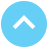# Impact of cancellation of “Hedges” (gold forward sales) by Barrick

Posted On Friday, Sep 25, 2009

Barrick had forward sales of 9.5 million ounces. At \$1000 per ounce gold price, Barrick has a mark to market loss of \$5.6 billion dollars.
This means that it’s per ounce loss approximately works out to \$590 per ounce.

This implies that the average price at which Barrick undertook its forward sales was approximately \$410 i.e. current price of \$1000 minus its current notional loss of \$590.

With cancelling these Hedges, Barrick is booking its loss of \$590.
But, at the same time, it frees its entire production to sell at spot prices as and when it produces.

Therefore, at any price above \$1000, Barrick stands to gain.

Different scenarios of Barrick’s profit / losses depending on gold prices:

In all the calculations below, we have assumed Barrick’s total cash cost of production to be \$410 per ounce. [Barrick’s total cash cost for Q209 was \$452 an ounce which in the future is likely to decline as new low cost mines start production. Therefore, we assume that his total cash cost to be approximately in the \$400-425 range.]

If the gold price remains constant at \$1000:

• Selling price = \$1000
• Loss o/s = \$590
• Net inflow = \$410

From this he needs to subtract his cash cost of production which is currently around \$410. On a net basis he earns no profit.

If prices increase to \$1100:

• Selling price = \$1100
• Loss o/s = \$590
• Net inflow = \$510

From this he needs to subtract his cash cost of production which is currently around \$410. On a net basis he earns a profit of \$100.

If prices fall to \$900:

• Selling price = \$900
• Loss o/s = \$590
• Net inflow = \$310

From this he needs to subtract his cash cost of production which is currently around \$410. On a net basis he incurs a loss of -\$100.

Barrick is booking a loss of \$590 assuming that the price won’t go below \$1000. As the price decreases below \$1000, the company will start incurring losses to that magnitude.
And any price above \$1000 will fetch gains for the company.

From a theoretical perspective, Barrick’s maximum loss of cancelling these hedges can be \$590. This is the maximum risk that Barrick is taking by cancelling the transaction on the main underlying assumption that prices will stay above \$1000. Barrick would be expecting some reward on account of taking the risk.
To calculate, the required gold price to gain a profit equivalent to the maximum loss that Barrick can have would be equal to the current gold price plus the maximum loss. This would translate to \$1000 current price + max loss of \$590 which is equivalent to \$1590.

View All

## Get In Touch

Take small steps to achieve big dreams! Start your investment journey today!